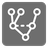On the same path
Simple
English RU

You have to write a function that receives a tree – potentially large but finite – and a list of pairs of tree's nodes.
For each pair, you must determine if the two nodes are on a same path (True) in the tree or not (False), then return an iterable/list of these booleans.

Input: two arguments:

• a tree as a tuple (a node, list of sub-trees)
• a list of pairs (the tuples of two nodes)

Output: An iterable/list of booleans.
Example:

```on_same_path(